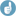## Precision of PowerTOST [Power / Sample Size]

Dear Detlew,

thank you for clarification
I tried to figure out the precision of PowerTOST estimations with the following code:
 library("ggplot2") library("PowerTOST") reps <- 1E6 scABELdata <- data.frame(rep = 1:reps) for(i in 1:reps){   set.seed(i)   scABELdata$power[i] <- power.scABEL(CV=0.8, n=54, theta0=0.95, design="2x3x3", nsims=10000, setseed=F) } ggplot(scABELdata, aes(power))+ geom_density(fill = 2, alpha = 0.3)+ theme_bw() + ggtitle(sprintf("%d reps: Mean is %.4f, SD is %.4f; %.2f %% obs are less than 0.8", reps, mean(scABELdata$power), sd(scABELdata$power), sum(scABELdata$power<0.8)/length(scABELdata\$power)*100))

please correct if it is wrong.
The resulted plot:BAsed on the mean and se calculated from sd and n=20 I would say that sample mean 80% in PowerTOST is a real unfortune❝ BTW: Both Laszlos recommend to use PowerTOST if they were asked.

Fully agree and thanks again for this wonderful package.

Kind regards,
MittyriIng. Helmut Schütz